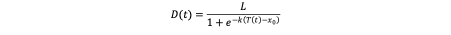The economic impact of climate change is often quantified as a fractional reduction in global or regional annual income, GDP, or GDP growth. This fractional reduction in GDP is estimated for multiple temperature values such as 2°C or 4°C warming. This relationship between the reduction in GDP and temperature is represented by a “damage function”, which maps temperature increase to estimated damage values.

Such estimates span a wide variety of values and forms. For instance, William Nordhaus, the Nobel laureate economist who pioneered the studies on the economic impact of climate change, used a quadratic function form which yields very low damage values for low temperatures, and estimated 2.1% of global income to be lost at a 3 °C warming, and 8.5% loss at a 6 °C. Other economists, such as Burke et al., estimated a rapid increase in damage even at low temperatures.

To account for the variety of damage function estimates and to allow for easily understandable user inputs, the damage function is formulated as a logistic (S-shaped) curve in En-ROADS. The equation below defines this logistic damage curve, where D(t) and T(t) are the damage and temperature increase over time, respectively. The constants L, and x0 refer to the maximum damage, steepness of the curve and the inflection point, respectively.The sliders Reduction in GDP at 2°C from climate impacts and Maximum reduction in GDP correspond to D(2) and L in this formulation.  Any and D(2) given by the users is used to calculate the parameters k and x0, hence the damage function for the temperature trajectory in current scenario.

This logistic damage function can replicate the estimates found in the literature as close as possible with certain input values. We chose four of these estimates, namely Nordhaus (2017), Weitzman (2012), Dietz and Stern (2015), and Burke et al. (2015), and fitted the logistic curve to these estimates using a least squares calibration approach. The resulting values for the two En-ROADS assumptions are listed in the table below, which can also be selected directly using the preset feature in the Assumptions view.